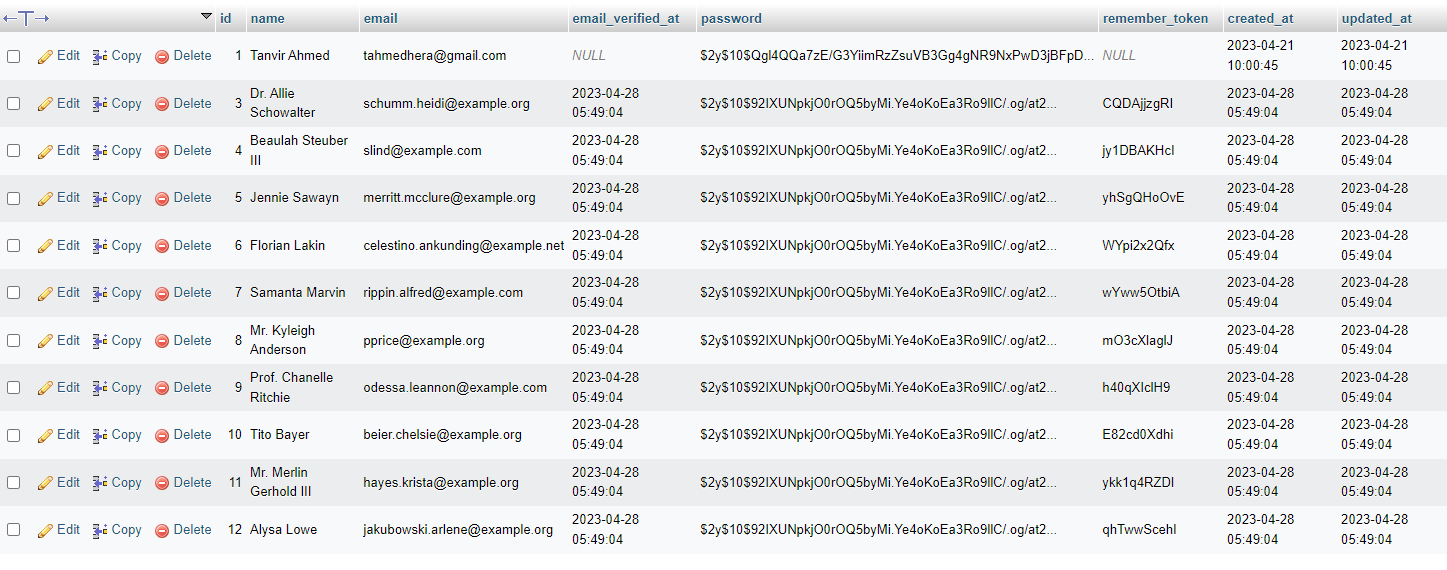# Laravel 10 - How to Generate Fake Data with Laravel's Faker LibraryMay 01, 2023 · Article · 3 min, 711 words

Hello Artisan, today I'll show you how to generate fake data using faker. Laravel's Faker library provides a simple way to generate fake data for your application. It can be used to generate fake names, addresses, phone numbers, dates, and much more. So, let's see how we can easily  create a set of fake data in our application.

Note: Tested on Laravel 10.8

1. Usage of Faker Library

## Usage of Faker Library

Faker can be useful for testing and development, or for creating sample data to show to clients or stakeholders. Let's see how we can easily create fake data. Faker give us the multiple helpful methods for generating the fake data. Let's see the available method first.

1. name(): Generates a random name.
2. firstName(): Generates a random first name.
3. lastName(): Generates a random last name.
4. email(): Generates a random email address.
6. phoneNumber(): Generates a random phone number.
8. city(): Generates a random city name.
9. state(): Generates a random state name.
10. postcode(): Generates a random postal code.
11. country(): Generates a random country name.
12. sentence(): Generates a random sentence.
13. paragraph(): Generates a random paragraph.
14. text(): Generates a random text.
15. randomElement(\$array): Returns a random element from the given array.
16. dateTime(\$max = 'now', \$timezone = null): Generates a random date and time.
17. numberBetween(\$min = 0, \$max = 2147483647): Generates a random number between the given range.
18. boolean(): Generates a random boolean value.

Lets' see how we can use these in our real life

``````\$faker = Faker\Factory::create();

dd(\$faker->name()); // will create a fake name``````

So as we see in the above example first we'll initialize a \$object of factory class and then we use a method called name() to generate a fake name. We can also get multiple names or countries as well. Let's look at the below example

``````\$faker = Faker\Factory::create();
\$names = [];
for (\$i = 0; \$i < 10; \$i++)
{
\$names[] = \$faker->country();
}
dd(\$names);  ``````

Which can give you the list of countries like the above image. An even we can use it to generate multiple row for a table. For this, first we've to create a faker first using the following command.

``php artisan make:factory FactoryName --model=ModelName``

For example we'll use the default UserFactory provided by Laravel. Just fire the below command in your terminal

``````php artisan tinker
App\Models\User::factory()->count(10)->create()``````

Add if you see in your table, you can see you've created 10 user in users table.So thus it'll help for creating dummy records and of course it'll help us in developments as well.

That's it for today. Hope this tutorial will be helpful in your upcoming projects. Thanks for reading. 🙂